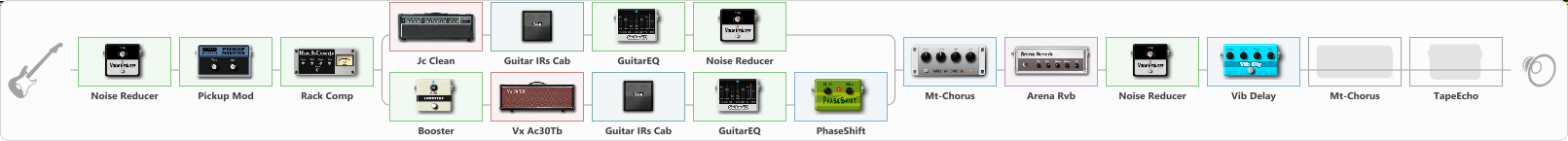# Best in my opinion clean tone

Discussion in 'ToneLib-GFX presets' started by ARSZ, Dec 28, 2021.

1. Best in my opinion clean tone

Preset name: Splitted clean

Effects chain:Effect: "Noise Reducer" (Dynamics / Filter), active - "yes"
{
"Sens" = 70
"Mode" = Hard
}

Effect: "Pickup Mod" (Dynamics / Filter), active - "yes"
{
"Mode" = Humbacker->Single
"Tone" = 39
}

Effect: "Rack Comp" (Dynamics / Filter), active - "yes"
{
"Threshold (dB)" = -11
"Ratio" = 16
"Attack" = Slow
"Release (ms)" = 843
"Knee" = 28
"Level (dB)" = 1
}

Effect: "Splitter" (Dynamics / Filter)
{
"A-Bypass" = Off
"A-Pan" = -26
"A-Level" = 76
"B-Bypass" = Off
"B-Pan" = 51
"B-Level" = 53

'A' branch:
{

Effect: "Jc Clean" (Amp simulators), active - "yes"
{
"Gain" = 35
"Bass" = 69
"Middle" = 63
"Treble" = 66
"Presence" = 85
"Master" = 50
"Level (dB)" = 4
}

Effect: "Guitar IRs Cab" (Cabinets), active - "yes"
{
"Model" = Fender Deluxe Rev (1x12")
"Mic Position" = Middle
"Mic Distance" = Middle
"Low Cut (Hz)" = 0
"Hi Cut (kHz)" = 20.0
"Mix" = 100
"Level (dB)" = 4
}

Effect: "GuitarEQ" (Dynamics / Filter), active - "yes"
{
"160 Hz" = 3
"400 Hz" = 3
"800 Hz" = 2
"1.6 kHz" = -7
"3.2 kHz" = -2
"6.4 kHz" = -5
"12 kHz" = -10
"Level (dB)" = 0
}

Effect: "Noise Reducer" (Dynamics / Filter), active - "yes"
{
"Sens" = 51
"Mode" = Hard
}
}
'B' branch:
{

Effect: "Booster" (Dynamics / Filter), active - "yes"
{
"Gain" = 36
}

Effect: "Vx Ac30Tb" (Amp simulators), active - "yes"
{
"Gain" = 50
"Bass" = 50
"Middle" = 50
"Treble" = 50
"Presence" = 50
"Master" = 50
"Level (dB)" = 0
}

Effect: "Guitar IRs Cab" (Cabinets), active - "yes"
{
"Model" = Fender Twin Rev (2x12")
"Mic Position" = Middle
"Mic Distance" = Middle
"Low Cut (Hz)" = 0
"Hi Cut (kHz)" = 20.0
"Mix" = 100
"Level (dB)" = 4
}

Effect: "GuitarEQ" (Dynamics / Filter), active - "yes"
{
"160 Hz" = -7
"400 Hz" = -5
"800 Hz" = -1
"1.6 kHz" = -7
"3.2 kHz" = -2
"6.4 kHz" = -5
"12 kHz" = -10
"Level (dB)" = 0
}

Effect: "PhaseShift" (Modulation / Sfx), active - "yes"
{
"Shift" = 71
"Mix" = 100
}
}
}

Effect: "Mt-Chorus" (Modulation / Sfx), active - "yes"
{
"Speed" = 5.7
"Depth" = 23
"Time" = 2.5
"Mix" = 23
}

Effect: "Arena Rvb" (Reverberation), active - "yes"
{
"Time" = 8.0
"PreDelay" = 33
"LoDamp" = 29
"HiDamp" = 35
"Mix" = 60
}

Effect: "Noise Reducer" (Dynamics / Filter), active - "yes"
{
"Sens" = 50
"Mode" = Soft
}

Effect: "Vib Delay" (Modulation / Sfx), active - "yes"
{
"Speed" = 4.2
"Depth" = 38
"Feedback" = 28
"Time" = 5.7
"Mix" = 50
"Mode" = 0
}

Effect: "Mt-Chorus" (Modulation / Sfx), active - "no"
{
"Speed" = 5.3
"Depth" = 92
"Time" = 2.5
"Mix" = 67
}

Effect: "TapeEcho" (Delay), active - "no"
{
"Time" = 624
"Feedback" = 50
"Tone" = 65
"LoDamp" = 19
"Mix" = 80
}

Note: You will need to download and install the ToneLib-GFX software to use the preset.

File size:
1.6 KB
Views:
2,324